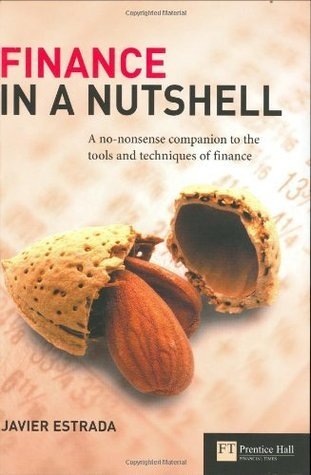# finance in a nutshellReview From User :

This book explains the mathematical foundations of major topics in finance. It took me six weeks to read it because it covers a lot of ground that I wanted to understand thoroughly.

There are plenty of equations, but the math isn't that difficult. There are a few infinite sums and some exponentials and logarithms. Of course some of the equations are very difficult to solve, but that doesn't mean the concepts they are expressing are that hard to understand.

The book is divided into brief, stand alone chapters that cover a major concept. This has the advantage of allowing the reader to just read what is interesting and jump from topic to topic in any order. The last few chapters provide an overview of statistics that are a good review before reading other sections.

I originally picked up this book because I was curious about how the price of a stock can be estimated. I've held stock in various companies that I've worked for, and I've always wondered how much it was really worth. ("Whatever someone is willing to pay for it," is the standard answer. Correct I suppose, but not very rigorous.) This book took me through dividend discount models, then cash flow-based models, then reverse valuation models, and finally relative valuation models. Each concept was simply stated in prose and the precisely stated with math.

After the sections on equity valuation I read straight through from the beginning. The only parts of the thirty chapters that I didn't find that interesting were futures and forwards and currency exchange.

How practical this knowledge is I can't say. I'm definitely not about to hedge my 401(k) with futures contracts any time soon. Also, I tried matching the value of GOOG options on the open market with my Black-Sholes calculations, but I either made a mistake in my spreadsheet or there is a major investment opportunity out there.

But then again now that I understand the basic math behind say, portfolio optimization, I'll get much more out of personal finance books like The Intelligent Asset Allocator (which I'm reading next).

At the end of each chapter are sample exercises, which I didn't take the time to work through. Also included in each chapter is a section that shows how to implement that chapter's calculations in Excel. I have a lot more appreciation for Excel after reading this book.

I would recommend this book to anyone with a mathematical bent and an interest in finance.

Media Size : 2.5 MB